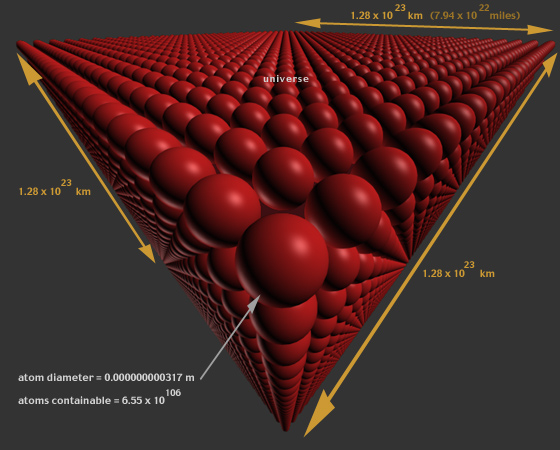`e v e r y  -  o b j e c t  `

The number of possible objects is determined by calculating the total number of atoms which could be contained in the universe, followed by calculating the number of possible molecular structures of these atoms, each structure having a different arrangement of the 109 elements at all locations in the universe. every-object determines the number of possible molecular structures of the universe with each structure viewed as a single object.

diameter of smallest atom (Helium) = .000000000062 m
diameter of largest atom (Cesium) = .000000000596 m
average size of atom = .000000000317 m = .000000000000317 km
molecule = substance comprised of 1 or more atoms
size of universe = 13500000000 light years
speed of light = 300000 km per second (186000 miles per second)
speed of light = 9467077824000 km per year (5869588250900 miles per year)size of universe (size of universe in light years x speed of light)
= 1.28 x 10^23 km (7.94 x 10^22 miles)

dimensions in universe = 3

 X size = 1.28 x 10^23 km (7.94 x 10^22 miles) Y size = 1.28 x 10^23 km (7.94 x 10^22 miles) Z size = 1.28 x 10^23 km (7.94 x 10^22 miles)number of possible atoms in one dimension = a a = (1.28 x 10^23 / .000000000000317 km) = 4.03 x 10^35number of atoms containable in universe (c) = a^3 = (4.031 x 10^35) ^3 = 6.55 x 10^106 number of elements = v = 109e = v^c every-object = e = 109^(6.55 x 10^106) possible objectssample molecular structure